Mobile QR CodeAnnealing, dielectric, FinFET, hot-carrier injection (HCI), punch-through, reliability, logic transistors

I. INTRODUCTION

As scaling down of complementary-metal-oxide semiconductors (CMOS) continues device reliability degradation is becoming a serious problem. Physical scaling down of gate length ($\textit{L}_{\mathrm{G}}$) and equivalent-oxide-thickness (EOT) have always been forwarded compared with supplied voltage ($\textit{V}_{\mathrm{DD}}$) scaling. In this context, the concern of hot-carrier injection (HCI), which stems from lateral high drain electric field, is important for device reliability. HCI increases the threshold voltage ($\textit{V}_{\mathrm{TH}}$) and subthreshold swing (\textit{SS}), as hot-carriers are captured in the gate dielectric or physically damage the SiO$_{2 }$$/ Si$ interface. Hence, HCI gives rise to $\textit{V}_{\mathrm{TH }}$mismatch in circuits, increases static power consumption, and reduces on-state current ($\textit{I}_{\mathrm{ON}}$) of field-effect-transistors (FETs) (1,2). To avoid the above-mentioned reliability concerns, applying a lightly doped drain (LDD), using gas annealing (FGA), and even applying high-pressure deuterium (HPD) annealing are preferred (3,4). Moreover, recently, a novel type of annealing called electro-thermal annealing (ETA) has been introduced to prolong device lifetime damaged by HCI. ETA generates localized Joule heat at a transistor level and thermally cures the damaged gate dielectrics, such as the gate oxide and gate spacer (5). Compared with conventional annealing processes such as FGA and HPD, ETA shows faster annealing speed, higher temperature, and better annealing selectivity.

However, even though device reliability and lifetime can be improved by ETA, additional power consumption is required for the annealing configurations. For example, the power consumption to trigger one iteration of ETA is quite high (a few mill-watts) due to high temperature Joule heat generation. Hence, development of ETA with lower power consumption is preferred for better practicality and applications, but related research has been modest until now.

In this work, device design guidelines are investigated to pursue better power efficiency of ETA. Simulation studies on device geometric sizes and materials have been performed. First, impact of device geometry, such as $L_G$ and channel width ($W_{NW}$), are discussed to understand scaling dependency. After that, dielectric layers such as gate spacers or buried dielectrics inserted on substrates are discussed in terms of heat sink and thermal isolation properties. It is possible that power consumption during ETA can be further reduced without great modification of device structure or materials.

Fig. 1. Schematic of device for SOI FinFET simulations (a) Top-view image of the device, (b) Cross-sectional view image of the device along the x-x' direction.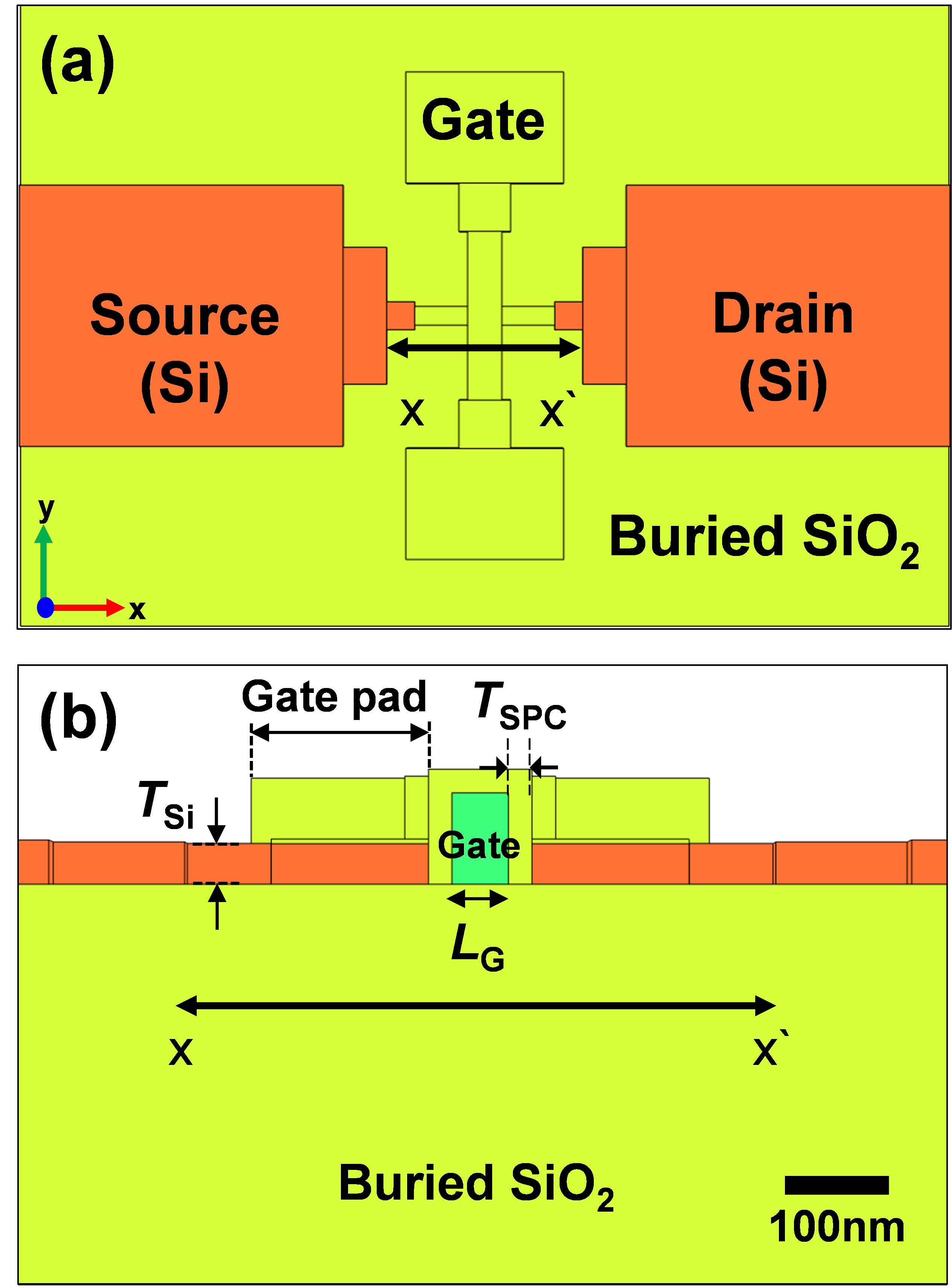II. Experimental Details

A tri-gate FinFET fabricated on SOI was simulated as a test vehicle. Punch-through annealing configuration was simulated among the other annealing configurations such as those using gate-to-gate (6) or forward bias current (7). Device structure and material, and the geometry of the SOI FinFET were taken from previous work (8). Detailed device fabrication process and transmission electron microscope (TEM) images are also included in the paper.

Fig. 1 shows a schematic of a tri-gate FinFET built on a BOX of 400 nm. The top silicon thickness ($T_{Si}$), channel width ($W_{NW}$), and $L_G$, of the device were 50 nm, 50 nm, and 60 nm, respectively. The gate spacer thickness ($T_{SPC}$) was 25 nm and was composed of $SiO_2$. Detailed device structure and material information in our simulations are summarized in Table 1.

Table 1. Device dimensions and material parameters for 3-dimensional simulations

 Dimension Material Thermal conductivity [W/m∙K] Gate Length, $L_G$ [nm] 60 Poly-Si 31.2 Gate Thickness [nm] 100 Spacer Thickness, $T_{SPC}$ [nm] 25 $SiO_2$ 1.25 Gate Dielectric Thickness [nm] 5 $SiO_2$ 1.25 Channel Thickness, $T_{Si}$ [nm] 50 Si 149 Channel Width, $W_{NW}$ [nm] 50 Buried Oxide Thickness [nm] 400 $SiO_2$ 1.25

Fig. 2. Measured $\textit{I}_{\mathrm{D}}$-$\textit{V}_{\mathrm{G}}$ characteristic of SOI FinFET.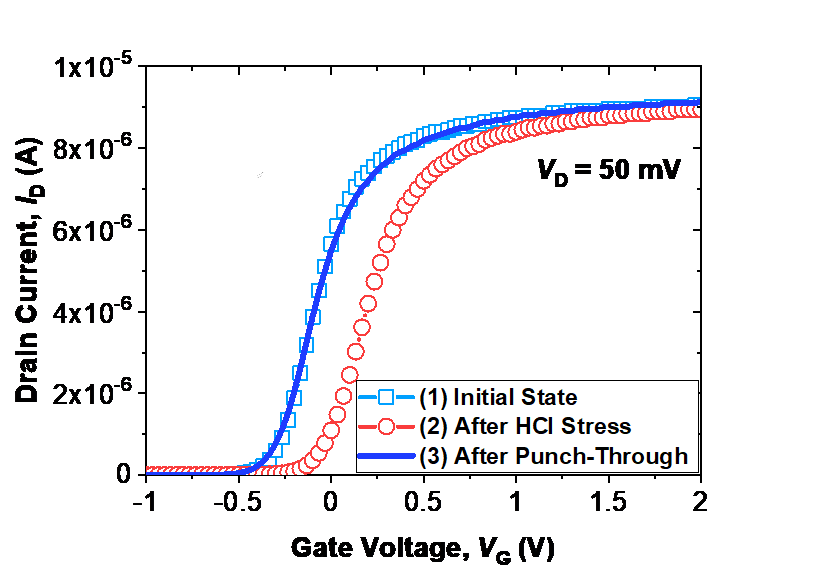III. Results and Discussion

Fig. 2 shows measured electrical I-V characteristic of an SOI FinFET. The DC characteristic was measured using a B1500A parameter analyzer at room temperature (9). After measurement of the initial state (e.g., initial state without stress), HCI stress at $V_{G}$ = 2 V and $V_{D}$ = 4 V was intentionally applied for 40 s. After the stress, degradation of the transconductance ($g_{m, max}$), SS, and $V_{TH}$ were observed at levels of 13 %, 38 %, and 27 %, respectively. After that, bias conditions at $V_{G}$ = 0.5 V and $V_{D}$ = 5.4 V were applied for 100 μs to activate punch-through annealing (Table 2).

After ETA, aged device characteristics with respect to $g_{m, max}$, SS, and $V_{TH}$ recovered by 93%, 90%, and 92%, respectively, compared to the initial state (Table 3). These facts show that both electrons trapped in gate dielectric and physical damage at $SiO_2$ $/ Si$ interface were effectively cured by the Joule heat generated during ETA. Fig. 3(a) shows simulated heat distribution profile during ETA by punch-through current. The Joule heating model in heat transfer module of COMSOL was utilized for 3-D thermal profiling. Moreover, during the simulation, environment condition and heat transfer coefficient (h) were assumed to be air and 10 W/m$^{2}$K, respectively. Generated Joule heat during ETA was well confined in SOI FinFET because of low thermal conductivity (1.2W/m∙K) of buried $SiO_2$ layer. Fig. 3(b) shows extracted temperature of the FinFET after reaching steady-state. It was confirmed that most of the heat during ETA was concentrated at the source/drain (S/D) extension where gate heat sink cannot affect (10).

Table 2. Bias conditions for punch-through current based ETA

 Bias Conditions Gate Voltage, $V_{G}$ 0.5 V Source Voltage, $V_S$ 0 V Drain Voltage, $V_D$ 5.4 V Drain Current, $I_D$ 670 μA Power Consumption, P = $V_D$ ˟ $I_D$ 3.6 mW Annealing Time, t 100 μs

Table 3. Device parameters extracted after HCI and ETA

 Initial State Hot-Carrier Injection Punch-Through Annealing $g_{m,max}$ (μA/V) 4.08 μA/V (100 %) 3.52 μA/V (86.4 %) 3.81 μA/V (93.4 %) SS (mV/dec) 133 mV/dec (100 %) 184 mV/dec (61.7 %) 146 mV/dec (90.3 %) $V_{TH}$ (V) - 0.327 V (100 %) - 0.089 V (72.7 %) -0.352 V (92.4 %)

Fig. 3. (a) Simulated heat distribution profile during ETA at $\textit{V}_{\mathrm{G}}$ = 0.5 V, $\textit{V}_{\mathrm{S}}$ = 0 V, and $\textit{V}_{\mathrm{D}}$ = 5.4 V, (b) Extracted temperature along the channel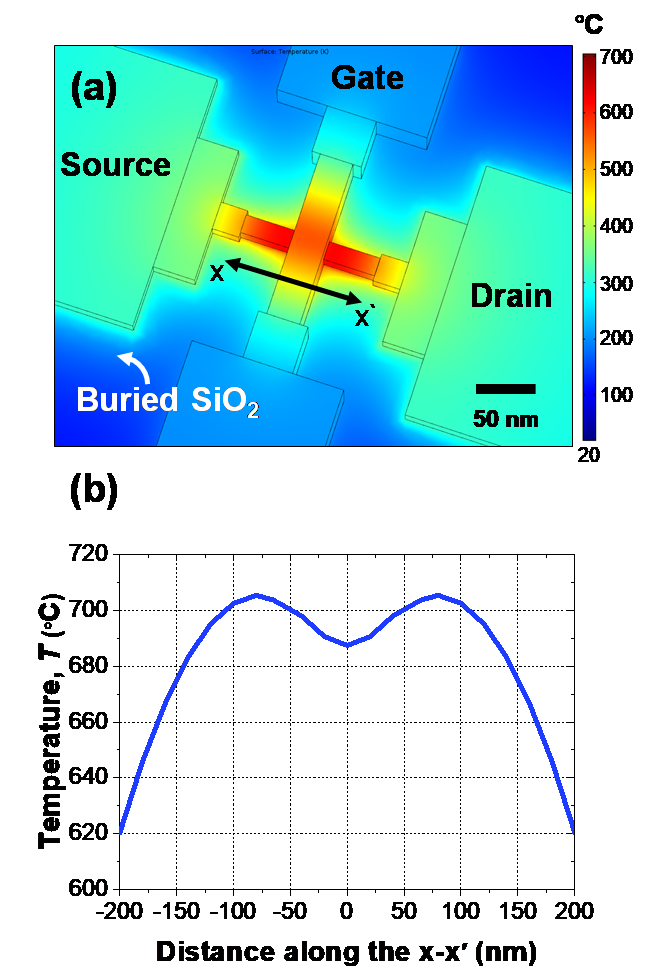Fig. 4. Extracted maximum temperature of punch-through current based ETA with various (a) $\textit{L}_{\mathrm{G}}$, (b) $\textit{W}_{\mathrm{NW}}$ under the same bias and power consumption.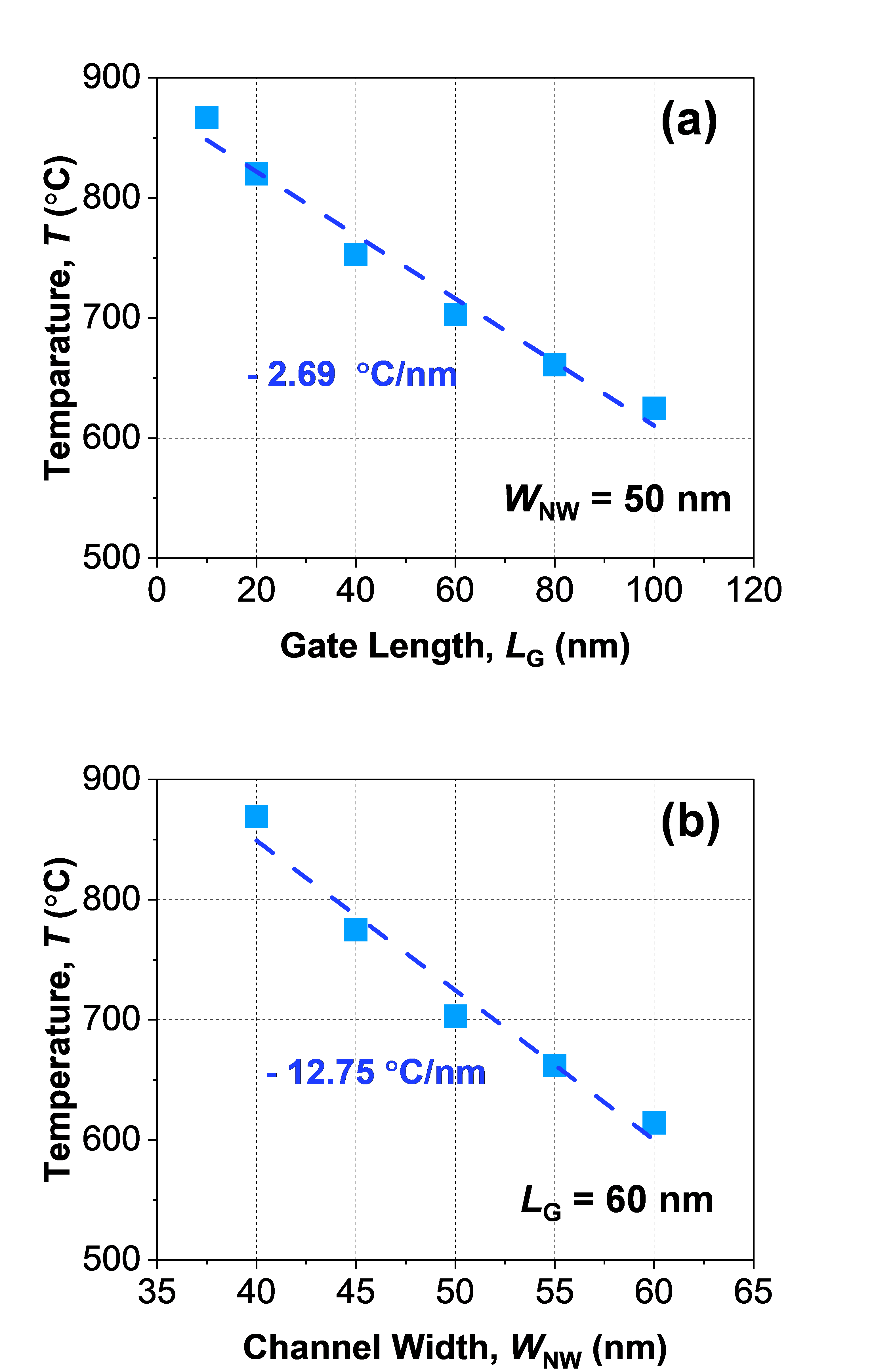Fig. 4 shows the extracted temperature with assumption of $L_G$ and $W_{NW}$ scaling. As $L_G$ decreases, the area of the gate heat sink decreases, and hence the Joule heat temperature increases. The extracted quantitative changing of temperature was - 2.69 °C / nm, as shown in Fig. 4(a).

Similarly, when $W_{NW}$ gradually dropped from 60 nm to 40 nm, the temperature of the device increased to - 12.75 °C / nm because of the reduced heat dissipation through the channel fin (11) (Fig. 4(b)). Hence, it can be concluded that the temperature generated during ETA can be raised under the same power consumption when LG or $W_{NW}$ scale down. In other words, power consumption required to activate ETA can be lowered by increasing the annealing efficiency. Especially, it should be noted that temperature change by $W_{NW}$ scale-down is about 5 times greater than in the case of $L_G$. This fact suggests that scaling down of $W_{NW}$ is much effective at improving ETA efficiency than scaling down of $L_G$. Moreover, considering there are several dielectrics in the SOI FinFET such as the gate dielectric, gate spacer, and buried dielectric layer, it is possible to improve annealing efficiency further.

Fig. 5. (a) Extracted maximum temperature with various gate spacer materials and thicknesses, (b) Temperature versus power consumption with various gate spacer materials.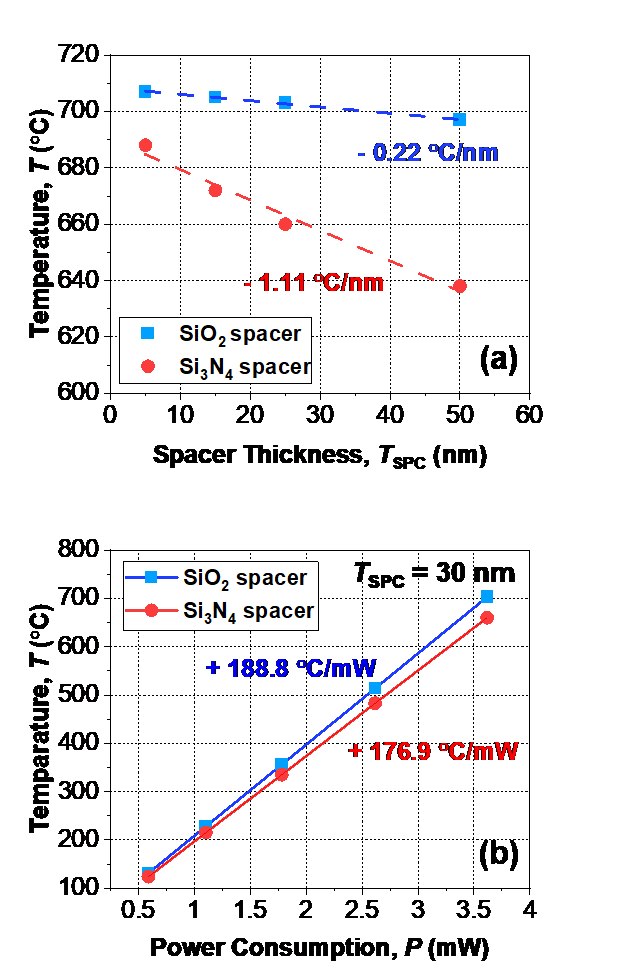Fig. 5(a) shows extracted temperature with various thicknesses of gate spacer ($T_{SPC}$). It was observed that the maximum temperature decreased as $T_{SPC}$ became thicker because a thicker $T_{SPC}$ has wider heat dissipation area (the interface between the S/D and the gate) than a thinner $T_{SPC}$. In the same vein, the gate spacer material with high thermal conductivity ($Si_{3}N_{4}$ ~ 40 W/m∙K) showed lower temperature than the $SiO_2$ gate spacer. Moreover, temperature changes of $Si_{3}N_{4}$ and $SiO_2$ as $T_{SPC}$ were - 0.22 °C / nm and - 1.11 °C / nm, respectively. Based on these facts, power consumption to generate identical temperature during ETA can be extracted, as shown in Fig. 5(b). The gate spacer composed of $SiO_2$ showed a value of + 188.8 °C / mW, while the gate spacer composed of $Si_{3}N_{4}$ showed a value of + 176.9 °C / mW.

Fig. 6. (a) Extracted maximum temperature with various buried dielectric materials and thicknesses, (b) Temperature versus power consumption with various buried dielectric materials.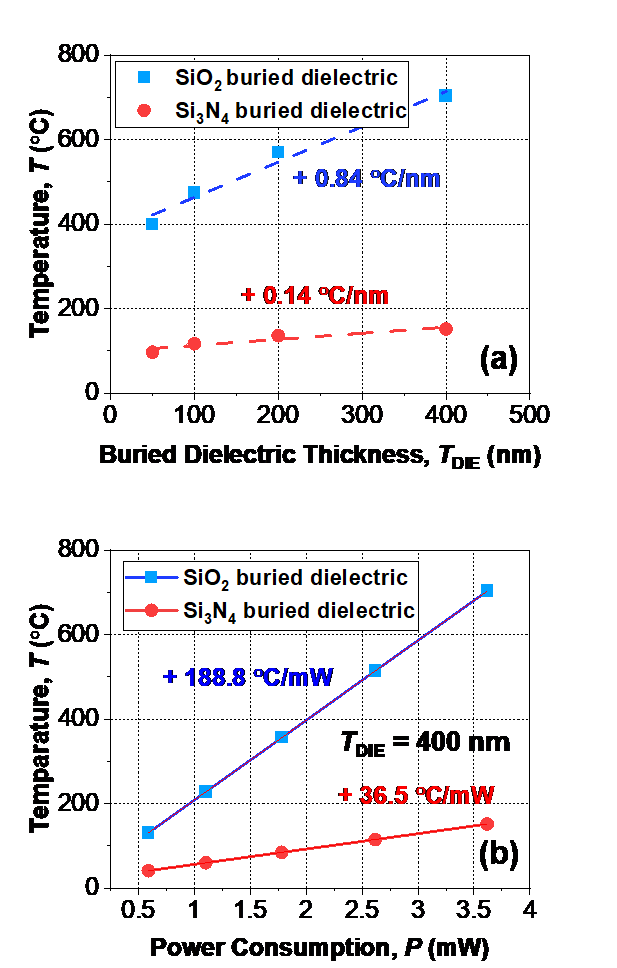This indicates that the gate spacer composed of $SiO_2$ has better power efficiency than that composed of $Si_{3}N_{4}$. It should be noted that thin-films composed of $SiO_2$ not only have two times lower dielectric constants, but are also preferred in terms of low power ETA over thin-films composed of $Si_{3}N_{4}$.

In addition, impact of buried dielectric layer, a representative material in SOI FinFETs, was investigated as shown in Fig. 6(a). Additional simulations were performed under assumption that $Si_{3}N_{4}$ layer can be replaced with buried $SiO_2$ (12,13). As the buried dielectric thickness ($T_{DIE}$) increases, the temperature during ETA also increases due to improved thermal isolation through the substrate.

Table 4. Summary of power efficiency during the ETA with respect to dielectric materials

 Gate Spacer Buried Dielectric $SiO_2$ $Si_{3}N_{4}$ $SiO_2$ +188 °C/mW +176 °C/mW $Si_{3}N_{4}$ +36 °C/mW +34 °C/mW (not shown)

When the buried dielectric was composed solely of $SiO_2$, extracted temperature sensitivity was + 0.84 °C / nm. On the other hand, when the substrate included $Si_{3}N_{4}$ instead of $SiO_2$, the sensitivity was just + 0.14 °C / nm. Fig. 6(b) shows power efficiency values of ETA with various buried dielectric materials. $SiO_2$ showed power efficiency (+ 188.8 °C / mW) five times better than that of $Si_{3}N_{4}$ (+ 36.5 °C / mW). It was confirmed that buried dielectric engineering played a great role in improving power efficiency during ETA. Based on these results, our recommendation to maximize the power efficiency is to apply $SiO_2$ for both gate spacer and buried dielectric, as summarized in Table 4.

Fig. 7 shows time-dependent characteristic with various thicknesses of dielectric layer composed of $SiO_2$. When there is no dielectric on substrate (bulk wafer), the temperature reached the saturation region within 3 ns owing to the superior thermal capacitance of silicon. However, as $T_{DIE}$ increases, the thermal time-constant during ETA with layer-widths of 50 nm, 100 nm, 200 nm of buried oxide were delayed to 25, 57, and 98 ns, respectively. The sensitivity of the thermal time-constant was extracted and found to be 0.48 ns / nm, as shown in Fig. 7(b). As a result, to maximize the annealing speed, it is preferred to include dielectric layers that are as thin as possible.

Fig. 7. (a) Time-dependent characteristic of temperature with various buried dielectric thicknesses (SiO$_{2}$), (b) Extracted thermal time-constant, which indicates time to reach saturation region.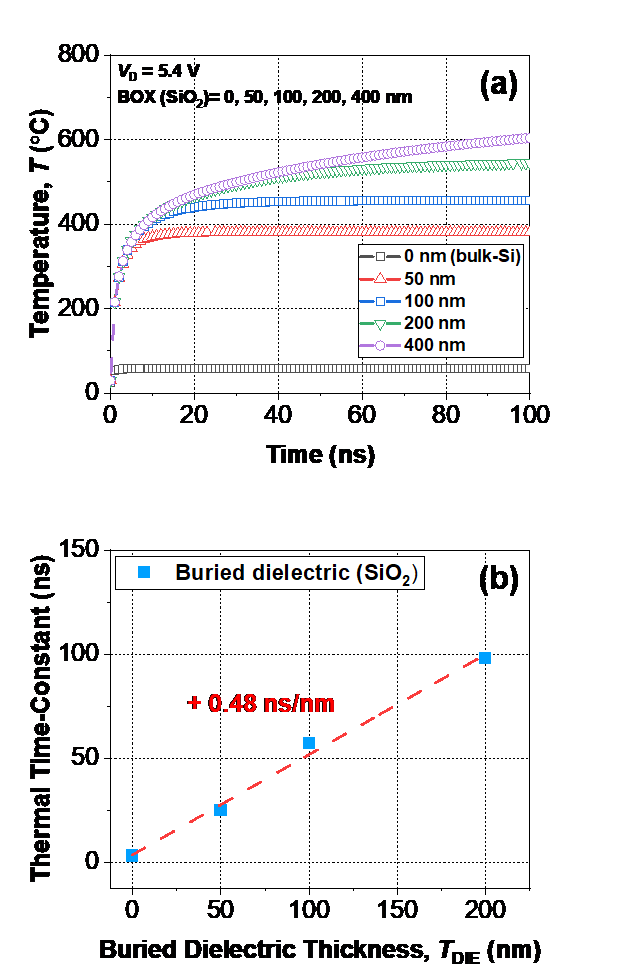IV. CONCLUSION

Degradation of device reliability stemming from hot-carrier injection (HCI) can be recoverable by using electro-thermal annealing (ETA) based on punch-through current. Impacts of device geometry structures and dielectric materials were discussed to minimize power consumption during ETA. As scaling down of device gate length ($\textit{L}_{\mathrm{G}}$) and channel width ($\textit{W}_{\mathrm{NW}}$) proceeded, temperature during ETA increased due to increased self-heating. Among various factors, $\textit{W}_{\mathrm{NW}}$ scaling dominated $\textit{L}_{\mathrm{G}}$ scaling. In addition, devices containing thicker buried dielectrics and thinner gate spacers showed better power efficiency than the other cases. Moreover, dielectrics with low thermal conductive materials were preferred because they maximized annealing effects under the same power consumption.

ACKNOWLEDGMENTS

This research was supported by Chungbuk National University Korea National University Development Project (2020).

REFERENCES

1
Hu C., Tam S. C., Hsu F.-C., Ko P.-K., Chan T.-Y., Terrill K. W., Feb 1985, Hot-electron-induced MOSFET degradation—Model, monitor, and improvement, IEEE Trans. Electron Devices, Vol. 32, No. 2, pp. 375-3852
Takeda E., Suzuki N., Apr 1983, An empirical model for device degradation due to hot-carrier injection, IEEE Electron Device Lett., Vol. 4, No. 4, pp. 111-1133
Onishi K., Kang C. S., Choi R., Cho H.-J., Gopalan S., Nieh R. E., Krishnan S. A., Lee J. C., Feb 2003, Improvement of surface carrier mobility of HfO2 MOSFETs by high-temperature forming gas annealing, IEEE Trans. Electron Devices, Vol. 50, No. 2, pp. 384-3904
Jo M., Chang M., Park H., Hwang H., Jun 2007, Improvement of hafnium oxide/silicon oxide gate dielectric stack qual,ity by high pressure D2O post deposition annealing, Jpn. J. Appl. Phys., Vol. 46, No. 22, pp. l531-L5335
Park J.-Y., Moon D.-I., Lee G.-B., Choi Y.-K., Mar 2020, Curing of aged gate dielectric by the self-heating effect in MOSFETs, IEEE Trans. Electron Devices, Vol. 67, No. 3, pp. 777-7886
Moon D.-I., Park J.-Y., Han J.-W., Jeon G.-J., Kim J.-Y., Moon J.-B., Seol M.-L., Kim C.-K., Lee H. C., Meyyappan M., Choi Y.-K., Dec 2016, Sustainable electronics for nano-spacecraft in deep space missions, Tech. Dig. - Int. Electron Devices Meet. IEDM, pp. 3-77
Lee G.-B., Kim C.-K., Park J.-Y., Bang T., Bae H., Kim S.-Y., Ryu S.-W., Choi Y.-K., Aug 2017, A Novel Technique for Curing Hot-Carrier-Induced Damage by Utilizing the Forward Current of the PN-Junction in a MOSFET, IEEE Electron Device Lett., Vol. 38, No. 8, pp. 1012-10148
Moon D.-I., Kim J.-Y., Jang H., Hong H.-J., Kim C. K., Oh J.-S., Kang M.-H., Kim J.-W., Choi Y.-K., Dec 2014, A novel FinFET with high-speed and prolonged retention for dynamic memory, IEEE Electron Device Lett., Vol. 35, No. 12, pp. 1236-12389
Park J.-Y., Hur J., Choi Y.-K., Feb 2018, Demonstration of a Curable Nanowire FinFET Using Punch-Through Current to Repair Hot-Carrier Damage, IEEE Electron Device Lett., Vol. 39, No. 2, pp. 180-18310
Jeon C.-H., Park J.-Y., Seol M.-L., Moon D.-I., Hur J., Bae H., Jeon S.-B., Choi Y.-K., Jun 2016, Joule heating to enhance the performance of a gate-all-around silicon nanowire transistor, IEEE Trans. Electron Devices, Vol. 63, No. 6, pp. 2288-229211
Ota K., Saitoh M., Tanaka C., Nakabayashi Y., Numata T., vol 59, Experimental Study of Self-Heating Effects in Trigate Nanowire MOSFETs Considering Device Geometry, IEEE Trans. Electron Devices12
Zhang Z.-X., Lin Q., Zhu M., Lin C.-L., Jul 2004, A new structure of SOI MOSFET for reducing self-heating effect, Ceramics Int, Vol. 30, No. 7, pp. 1289-129313
Roig J., Flores D., Vellvehi M., Rebollo J., Millan J., Jan 2002, Reduction of self-heating effect in SOIM devices, Microelectronics Rel, Vol. 42, No. 1, pp. 61-66Author

Dong-Woo Cha is currently pursuing the B.S. degree from the School of Electronics Engineering, Chungbuk National University, Cheongju, Republic of Korea.

His current research interests include the simulation of semiconductor devices.

Jun-Young Park received the B.S. degree from the School of Electrical and Electronic Engineering, Yonsei University, Seoul, Republic of Korea, in 2014, and the M.S. & Ph.D. degree from the Korea Advanced Institute of Science and Technology, Daejeon, Republic of Korea, in 2016 and 2020.

He is currently the Assistant Professor of School of Electronics Engineering, Chungbuk National University, Cheongju, Republic of Korea.

His current research interests include reliability of semiconductor devices.